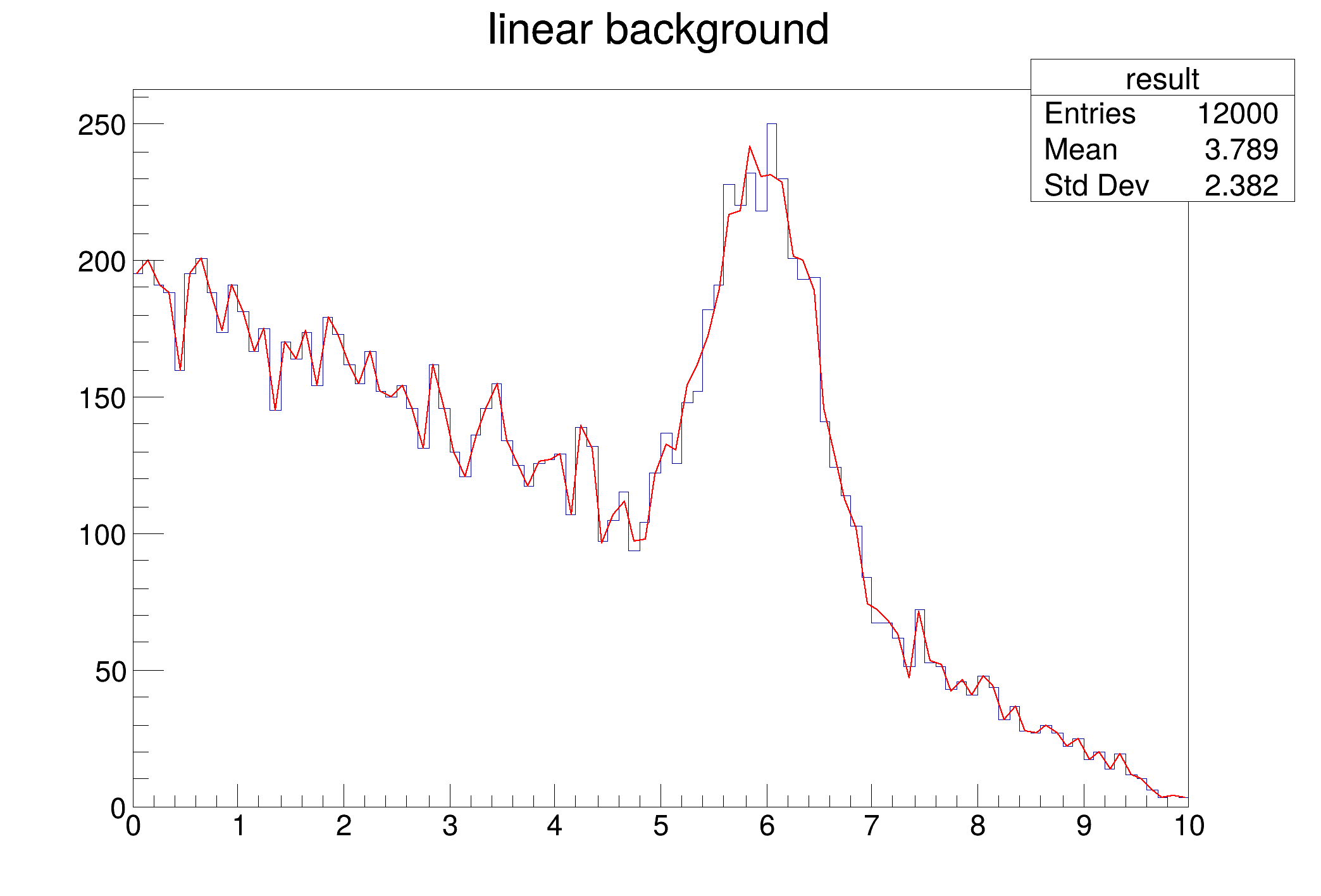ROOT   Reference Guide
Searching...
No Matches
fithist.C File Reference

## Detailed DescriptionExample of fit where the model is histogram + function****************************************
Chi2 = 7.84157
NDf = 96
Edm = 3.7399e-06
NCalls = 174
p0 = 156.638 +/- 5.91061 (limited)
p1 = 5.98183 +/- 0.0189043
p2 = -0.506659 +/- 0.0180481
p3 = 1.0003 +/- 0.0112955
#include <TF1.h>
#include <TFile.h>
#include <TH1F.h>
TH1F *background;
void histgen() {
//generate the histogram background and save it to a file
//background taken as linearly decreasing
TF1 f1("f1","pol1",0,10);
f1.SetParameters(5,-0.5);
TH1F h("background","linear background",100,0,10);
h.FillRandom("f1",10000);
TFile f("background.root","recreate");
//save the background histogram
h.Write();
//superimpose a Gaussian signal to the background histogram
TF1 f2("f2","gaus",0,10);
f2.SetParameters(1,6,0.5);
h.FillRandom("f2",2000);
h.SetName("result");
h.Write();
}
double ftotal(double *x, double *par) {
double xx = x;
int bin = background->GetXaxis()->FindBin(xx);
double br = par*background->GetBinContent(bin);
double arg = (xx-par)/par;
double sr = par*TMath::Exp(-0.5*arg*arg);
return sr + br;
}
void fithist() {
//fit function ftotal to signal + background
histgen();
TFile *f = new TFile("background.root");
background = (TH1F*)f->Get("background"); //pointer used in ftotal
TH1F *result = (TH1F*)f->Get("result");
TF1 *ftot = new TF1("ftot",ftotal,0,10,4);
double norm = result->GetMaximum();
ftot->SetParameters(0.5*norm,5,.2,norm);
ftot->SetParLimits(0,.3*norm,norm);
result->Fit("ftot","b");
}
#define f(i)
Definition RSha256.hxx:104
#define h(i)
Definition RSha256.hxx:106
Option_t Option_t TPoint TPoint const char GetTextMagnitude GetFillStyle GetLineColor GetLineWidth GetMarkerStyle GetTextAlign GetTextColor GetTextSize void char Point_t Rectangle_t WindowAttributes_t Float_t Float_t Float_t Int_t Int_t UInt_t UInt_t Rectangle_t result
virtual Int_t FindBin(Double_t x)
Find bin number corresponding to abscissa x.
Definition TAxis.cxx:293
1-Dim function class
Definition TF1.h:213
virtual void SetParLimits(Int_t ipar, Double_t parmin, Double_t parmax)
Set lower and upper limits for parameter ipar.
Definition TF1.cxx:3499
virtual void SetParameters(const Double_t *params)
Definition TF1.h:649
A ROOT file is composed of a header, followed by consecutive data records (TKey instances) with a wel...
Definition TFile.h:54
1-D histogram with a float per channel (see TH1 documentation)}
Definition TH1.h:577
TAxis * GetXaxis()
Definition TH1.h:322
virtual Double_t GetBinContent(Int_t bin) const
Return content of bin number bin.
Definition TH1.cxx:5028
Double_t x[n]
Definition legend1.C:17
TF1 * f1
Definition legend1.C:11
Double_t Exp(Double_t x)
Returns the base-e exponential function of x, which is e raised to the power x.
Definition TMath.h:709

Definition in file fithist.C.